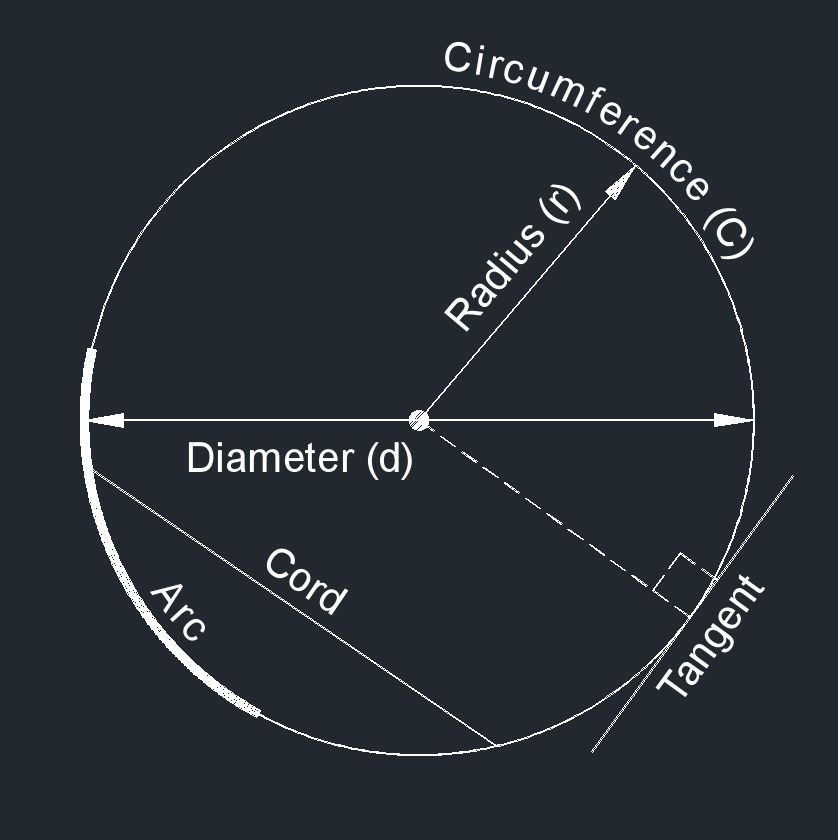# Area

Written by Jerry Ratzlaff on . Posted in Geometry

###Area, abbreviated as A, is the square units of a given plane.

## Formulas that use Area

 $$\large{ A = \pi \; r^2 }$$ (circle) $$\large{ A = \frac{ \pi \; d^2 }{ 4 } }$$ $$\large{ A = \frac{ C \; r }{ 2 } }$$ $$\large{ A = \frac{ C^2 }{ 4 \; \pi } }$$ $$\large{ A = \frac{F}{p} }$$ $$\large{ A = \frac{ Q }{ v } }$$ $$\large{ A = a_c^2 }$$ (cube face area) $$\large{ A = 6\;a_c^2 }$$ (cube surface face area) $$\large{ A = \pi \;a_e\; b_e }$$ (ellipse) $$\large{ A = \frac{F}{\sigma} }$$ (force) $$\large{ A = \frac{2 \; L}{ C_l \; \rho \; v^2} }$$ (lift force)

### Where:

$$\large{ A }$$ = area

$$\large{ C }$$ = circumference

$$\large{ a_c }$$ = cube edge

$$\large{ \rho }$$  (Greek symbol rho) = density

$$\large{ Q }$$ = flow rate

$$\large{ F }$$ = force

$$\large{ a_e }$$ = ellipse length semi-major axis

$$\large{ b_e }$$ = ellipse length semi-minor axis

$$\large{ C_l }$$ = lift coefficient

$$\large{ L }$$ = lift force

$$\large{ \pi }$$ = Pi

$$\large{ p }$$ = pressure

$$\large{ r }$$ = radius

$$\large{ \sigma }$$  (Greek symbol sigma) = stress

$$\large{ v }$$ = velocity

$$\large{ \sigma }$$  (Greek symbol sigma) = yield strength